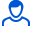Content: teorver_5595.doc (29.50 KB)

Positive responses: 0
Negative responses: 0

Refunds: 05595. x̅=17.7, n=57, σ=0.7. Find the confidence interval for the mathematical expectation a of a normally distributed quantitative attribute X of the general population with reliability γ=0.95, knowing the sample mean x̅в=17.7; sample size n=57 and standard deviation σ=0.7.
Detailed solution. Decorated in Microsoft Word 2003 (Quest decided to use the formula editor)
No feedback yet